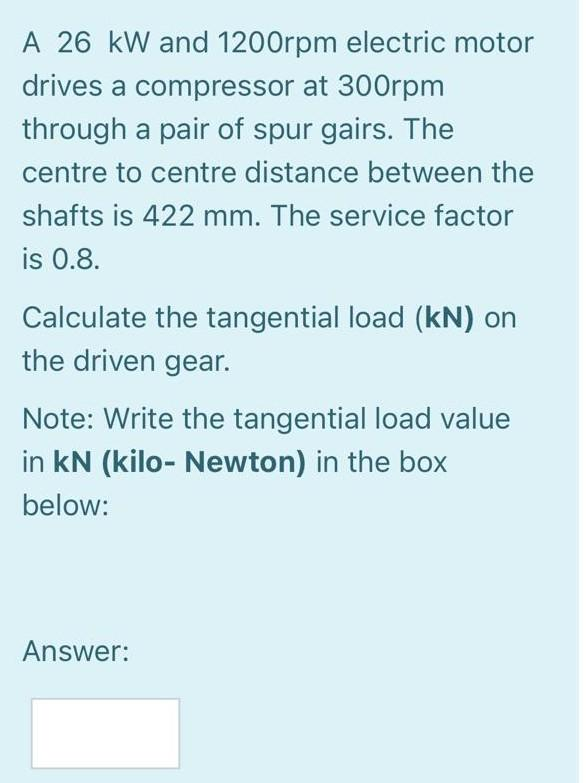# Question A 26 kW and 1200rpm electric motor drives a compressor at 300rpm through a pair of spur gairs. The centre to centre distance between the shafts is 422 mm. The service factor is 0.8. Calculate the tangential load (kN) on the driven gear. Note: Write the tangential load value in kN (kilo- Newton) in the box below: Answer:WE5K8T The Asker · Mechanical EngineeringTranscribed Image Text: A 26 kW and 1200rpm electric motor drives a compressor at 300rpm through a pair of spur gairs. The centre to centre distance between the shafts is 422 mm. The service factor is 0.8. Calculate the tangential load (kN) on the driven gear. Note: Write the tangential load value in kN (kilo- Newton) in the box below: Answer:
More
Transcribed Image Text: A 26 kW and 1200rpm electric motor drives a compressor at 300rpm through a pair of spur gairs. The centre to centre distance between the shafts is 422 mm. The service factor is 0.8. Calculate the tangential load (kN) on the driven gear. Note: Write the tangential load value in kN (kilo- Newton) in the box below: Answer: